# Image segmentation with U-Net

In image segmentation, every pixel of an image is assigned a class. Depending on the application, classes could be different cell types; or the task could be binary, as in “cancer cell yes or no?”. Area of application notwithstanding, the established neural network architecture of choice is U-Net. In this post, we show how to preprocess data and train a U-Net model on the Kaggle Carvana image segmentation data.

Daniel Falbel (RStudio)https://www.rstudio.com/ , Sigrid Keydana (RStudio)https://www.rstudio.com/
08-23-2019

Sure, it is nice when I have a picture of some object, and a neural network can tell me what kind of object that is. More realistically, there might be several salient objects in that picture, and it tells me what they are, and where they are. The latter task (known as object detection) seems especially prototypical of contemporary AI applications that at the same time are intellectually fascinating and ethically questionable. It’s different with the subject of this post: Successful image segmentation has a lot of undeniably useful applications. For example, it is a sine qua non in medicine, neuroscience, biology and other life sciences.

So what, technically, is image segmentation, and how can we train a neural network to do it?

## Image segmentation in a nutshell

Say we have an image with a bunch of cats in it. In classification, the question is “what’s that?”, and the answer we want to hear is: “cat”. In object detection, we again ask “what’s that”, but now that “what” is implicitly plural, and we expect an answer like “there’s a cat, a cat, and a cat, and they’re here, here, and here” (imagine the network pointing, by means of drawing bounding boxes, i.e., rectangles around the detected objects). In segmentation, we want more: We want the whole image covered by “boxes” – which aren’t boxes anymore, but unions of pixel-size “boxlets” – or put differently: We want the network to label every single pixel in the image.

Here’s an example from the paper we’re going to talk about in a second. On the left is the input image (HeLa cells), next up is the ground truth, and third is the learned segmentation mask.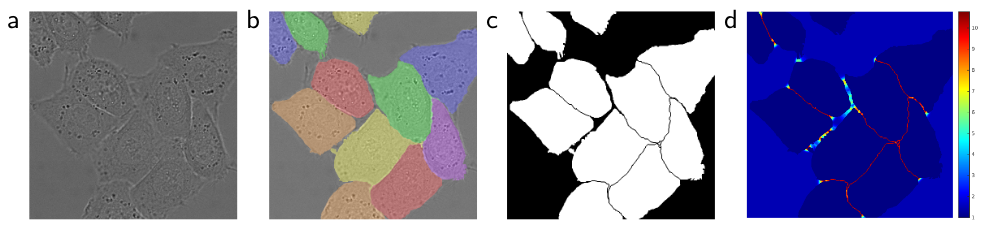Figure 1: Example segmentation from Ronneberger et al. 2015.

Technically, a distinction is made between class segmentation and instance segmentation. In class segmentation, referring to the “bunch of cats” example, there are two possible labels: Every pixel is either “cat” or “not cat”. Instance segmentation is more difficult: Here every cat gets their own label. (As an aside, why should that be more difficult? Presupposing human-like cognition, it wouldn’t be – if I have the concept of a cat, instead of just “cattiness”, I “see” there are two cats, not one. But depending on what a specific neural network relies on most – texture, color, isolated parts – those tasks may differ a lot in difficulty.)

The network architecture used in this post is adequate for class segmentation tasks and should be applicable to a vast number of practical, scientific as well as non-scientific applications. Speaking of network architecture, how should it look?

## Introducing U-Net

Given their success in image classification, can’t we just use a classic architecture like Inception V[n], ResNet, ResNext … , whatever? The problem is, our task at hand – labeling every pixel – does not fit so well with the classic idea of a CNN. With convnets, the idea is to apply successive layers of convolution and pooling to build up feature maps of decreasing granularity, to finally arrive at an abstract level where we just say: “yep, a cat”. The counterpart being, we lose detail information: To the final classification, it does not matter whether the five pixels in the top-left area are black or white.

In practice, the classic architectures use (max) pooling or convolutions with `stride` > 1 to achieve those successive abstractions – necessarily resulting in decreased spatial resolution. So how can we use a convnet and still preserve detail information? In their 2015 paper U-Net: Convolutional Networks for Biomedical Image Segmentation (Ronneberger, Fischer, and Brox 2015), Olaf Ronneberger et al. came up with what four years later, in 2019, is still the most popular approach. (Which is to say something, four years being a long time, in deep learning.)

The idea is stunningly simple. While successive encoding (convolution / max pooling) steps, as usual, reduce resolution, the subsequent decoding – we have to arrive at an output of size same as the input, as we want to label every pixel! – does not simply upsample from the most compressed layer. Instead, during upsampling, at every step we feed in information from the corresponding, in resolution, layer in the downsizing chain.

For U-Net, really a picture says more than many words: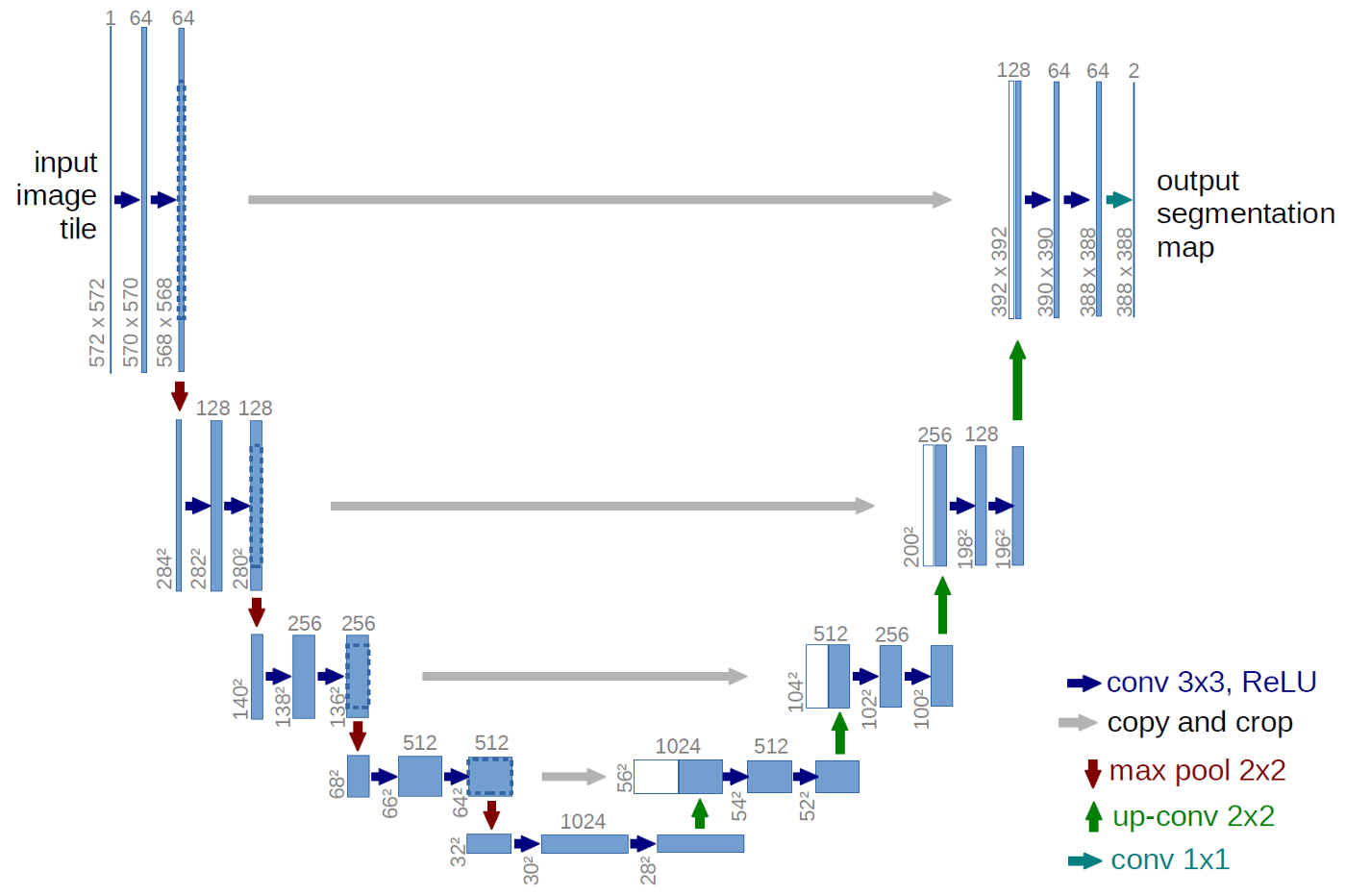Figure 2: U-Net architecture from Ronneberger et al. 2015.

At each upsampling stage we concatenate the output from the previous layer with that from its counterpart in the compression stage. The final output is a mask of size the original image, obtained via 1x1-convolution; no final dense layer is required, instead the output layer is just a convolutional layer with a single filter.

Now let’s actually train a U-Net. We’re going to use the `unet` package that lets you create a well-performing model in a single line:

``````
remotes::install_github("r-tensorflow/unet")
library(unet)

# takes additional parameters, including number of downsizing blocks,
# number of filters to start with, and number of classes to identify
model <- unet(input_shape = c(128, 128, 3))``````

So we have a model, and it looks like we’ll be wanting to feed it 128x128 RGB images. Now how do we get these images?

## The data

To illustrate how applications arise even outside the area of medical research, we’ll use as an example the Kaggle Carvana Image Masking Challenge. The task is to create a segmentation mask separating cars from background. For our current purpose, we only need `train.zip` and `train_mask.zip` from the archive provided for download. In the following, we assume those have been extracted to a subdirectory called `data-raw`.

Let’s first take a look at some images and their associated segmentation masks.

``````
# libraries we're going to need later
library(keras)
library(tfdatasets)
library(tidyverse)
library(rsample)
library(reticulate)

images <- tibble(
img = list.files(here::here("data-raw/train"), full.names = TRUE),
) %>%
sample_n(2) %>%

out <- magick::image_append(c(
magick::image_append(images\$img, stack = TRUE),
)
)``````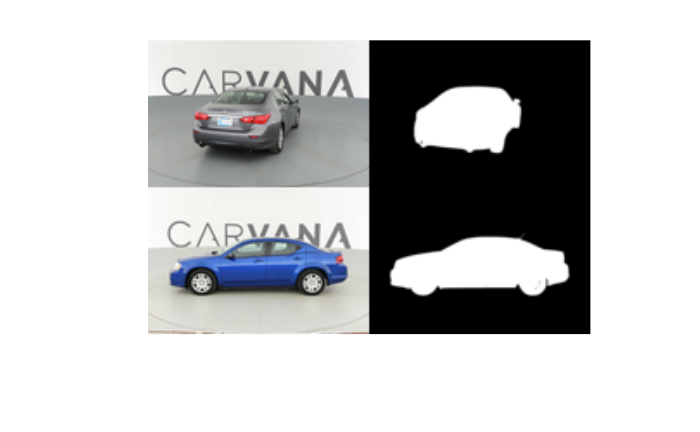The photos are RGB-space JPEGs, while the masks are black-and-white GIFs.

We split the data into a training and a validation set. We’ll use the latter to monitor generalization performance during training.

``````
data <- tibble(
img = list.files(here::here("data-raw/train"), full.names = TRUE),
)

data <- initial_split(data, prop = 0.8)``````

To feed the data to the network, we’ll use tfdatasets. All preprocessing will end up in a simple pipeline, but we’ll first go over the required actions step-by-step.

## Preprocessing pipeline

The first step is to read in the images, making use of the appropriate functions in `tf\$image`.

``````
training_dataset <- training(data) %>%
tensor_slices_dataset() %>%
dataset_map(~.x %>% list_modify(
# decode_jpeg yields a 3d tensor of shape (1280, 1918, 3)
# decode_gif yields a 4d tensor of shape (1, 1280, 1918, 3),
# so we remove the unneeded batch dimension and all but one
# of the 3 (identical) channels
))``````

While constructing a preprocessing pipeline, it’s very useful to check intermediate results. It’s easy to do using `reticulate::as_iterator` on the dataset:

``````
example <- training_dataset %>% as_iterator() %>% iter_next()
example``````
``````
\$img
tf.Tensor(
[[[243 244 239]
[243 244 239]
[243 244 239]
...
...
...
[175 179 178]
[175 179 178]
[175 179 178]]], shape=(1280, 1918, 3), dtype=uint8)

tf.Tensor(
[[


...
...
...


]], shape=(1280, 1918, 1), dtype=uint8)
``````

While the `uint8` datatype makes RGB values easy to read for humans, the network is going to expect floating point numbers. The following code converts its input and additionally, scales values to the interval [0,1):

``````
training_dataset <- training_dataset %>%
dataset_map(~.x %>% list_modify(
img = tf\$image\$convert_image_dtype(.x\$img, dtype = tf\$float32),
))``````

To reduce computational cost, we resize the images to size `128x128`. This will change the aspect ratio and thus, distort the images, but is not a problem with the given dataset.

``````
training_dataset <- training_dataset %>%
dataset_map(~.x %>% list_modify(
img = tf\$image\$resize(.x\$img, size = shape(128, 128)),
))``````

Now, it’s well known that in deep learning, data augmentation is paramount. For segmentation, there’s one thing to consider, which is whether a transformation needs to be applied to the mask as well – this would be the case for e.g. rotations, or flipping. Here, results will be good enough applying just transformations that preserve positions:

``````
random_bsh <- function(img) {
img %>%
tf\$image\$random_brightness(max_delta = 0.3) %>%
tf\$image\$random_contrast(lower = 0.5, upper = 0.7) %>%
tf\$image\$random_saturation(lower = 0.5, upper = 0.7) %>%
# make sure we still are between 0 and 1
tf\$clip_by_value(0, 1)
}

training_dataset <- training_dataset %>%
dataset_map(~.x %>% list_modify(
img = random_bsh(.x\$img)
))``````

Again, we can use `as_iterator` to see what these transformations do to our images:

``````
example <- training_dataset %>% as_iterator() %>% iter_next()
example\$img %>% as.array() %>% as.raster() %>% plot()``````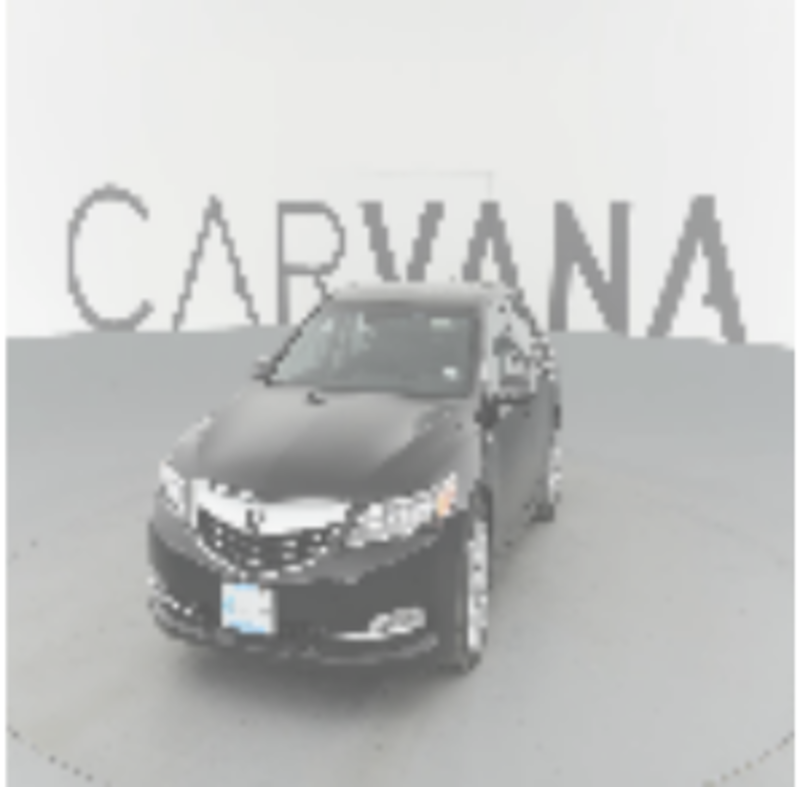Here’s the complete preprocessing pipeline.

``````
create_dataset <- function(data, train, batch_size = 32L) {

dataset <- data %>%
tensor_slices_dataset() %>%
dataset_map(~.x %>% list_modify(
)) %>%
dataset_map(~.x %>% list_modify(
img = tf\$image\$convert_image_dtype(.x\$img, dtype = tf\$float32),
)) %>%
dataset_map(~.x %>% list_modify(
img = tf\$image\$resize(.x\$img, size = shape(128, 128)),
))

# data augmentation performed on training set only
if (train) {
dataset <- dataset %>%
dataset_map(~.x %>% list_modify(
img = random_bsh(.x\$img)
))
}

# shuffling on training set only
if (train) {
dataset <- dataset %>%
dataset_shuffle(buffer_size = batch_size*128)
}

# train in batches; batch size might need to be adapted depending on
# available memory
dataset <- dataset %>%
dataset_batch(batch_size)

dataset %>%
# output needs to be unnamed
dataset_map(unname)
}``````

Training and test set creation now is just a matter of two function calls.

``````
training_dataset <- create_dataset(training(data), train = TRUE)
validation_dataset <- create_dataset(testing(data), train = FALSE)``````

And we’re ready to train the model.

## Training the model

We already showed how to create the model, but let’s repeat it here, and check model architecture:

``````
model <- unet(input_shape = c(128, 128, 3))
summary(model)``````
``````
Model: "model"
______________________________________________________________________________________________
Layer (type)                   Output Shape        Param #    Connected to
==============================================================================================
input_1 (InputLayer)           [(None, 128, 128, 3 0
______________________________________________________________________________________________
conv2d (Conv2D)                (None, 128, 128, 64 1792       input_1
______________________________________________________________________________________________
conv2d_1 (Conv2D)              (None, 128, 128, 64 36928      conv2d
______________________________________________________________________________________________
max_pooling2d (MaxPooling2D)   (None, 64, 64, 64)  0          conv2d_1
______________________________________________________________________________________________
conv2d_2 (Conv2D)              (None, 64, 64, 128) 73856      max_pooling2d
______________________________________________________________________________________________
conv2d_3 (Conv2D)              (None, 64, 64, 128) 147584     conv2d_2
______________________________________________________________________________________________
max_pooling2d_1 (MaxPooling2D) (None, 32, 32, 128) 0          conv2d_3
______________________________________________________________________________________________
conv2d_4 (Conv2D)              (None, 32, 32, 256) 295168     max_pooling2d_1
______________________________________________________________________________________________
conv2d_5 (Conv2D)              (None, 32, 32, 256) 590080     conv2d_4
______________________________________________________________________________________________
max_pooling2d_2 (MaxPooling2D) (None, 16, 16, 256) 0          conv2d_5
______________________________________________________________________________________________
conv2d_6 (Conv2D)              (None, 16, 16, 512) 1180160    max_pooling2d_2
______________________________________________________________________________________________
conv2d_7 (Conv2D)              (None, 16, 16, 512) 2359808    conv2d_6
______________________________________________________________________________________________
max_pooling2d_3 (MaxPooling2D) (None, 8, 8, 512)   0          conv2d_7
______________________________________________________________________________________________
dropout (Dropout)              (None, 8, 8, 512)   0          max_pooling2d_3
______________________________________________________________________________________________
conv2d_8 (Conv2D)              (None, 8, 8, 1024)  4719616    dropout
______________________________________________________________________________________________
conv2d_9 (Conv2D)              (None, 8, 8, 1024)  9438208    conv2d_8
______________________________________________________________________________________________
conv2d_transpose (Conv2DTransp (None, 16, 16, 512) 2097664    conv2d_9
______________________________________________________________________________________________
concatenate (Concatenate)      (None, 16, 16, 1024 0          conv2d_7
conv2d_transpose
______________________________________________________________________________________________
conv2d_10 (Conv2D)             (None, 16, 16, 512) 4719104    concatenate
______________________________________________________________________________________________
conv2d_11 (Conv2D)             (None, 16, 16, 512) 2359808    conv2d_10
______________________________________________________________________________________________
conv2d_transpose_1 (Conv2DTran (None, 32, 32, 256) 524544     conv2d_11
______________________________________________________________________________________________
concatenate_1 (Concatenate)    (None, 32, 32, 512) 0          conv2d_5
conv2d_transpose_1
______________________________________________________________________________________________
conv2d_12 (Conv2D)             (None, 32, 32, 256) 1179904    concatenate_1
______________________________________________________________________________________________
conv2d_13 (Conv2D)             (None, 32, 32, 256) 590080     conv2d_12
______________________________________________________________________________________________
conv2d_transpose_2 (Conv2DTran (None, 64, 64, 128) 131200     conv2d_13
______________________________________________________________________________________________
concatenate_2 (Concatenate)    (None, 64, 64, 256) 0          conv2d_3
conv2d_transpose_2
______________________________________________________________________________________________
conv2d_14 (Conv2D)             (None, 64, 64, 128) 295040     concatenate_2
______________________________________________________________________________________________
conv2d_15 (Conv2D)             (None, 64, 64, 128) 147584     conv2d_14
______________________________________________________________________________________________
conv2d_transpose_3 (Conv2DTran (None, 128, 128, 64 32832      conv2d_15
______________________________________________________________________________________________
concatenate_3 (Concatenate)    (None, 128, 128, 12 0          conv2d_1
conv2d_transpose_3
______________________________________________________________________________________________
conv2d_16 (Conv2D)             (None, 128, 128, 64 73792      concatenate_3
______________________________________________________________________________________________
conv2d_17 (Conv2D)             (None, 128, 128, 64 36928      conv2d_16
______________________________________________________________________________________________
conv2d_18 (Conv2D)             (None, 128, 128, 1) 65         conv2d_17
==============================================================================================
Total params: 31,031,745
Trainable params: 31,031,745
Non-trainable params: 0
______________________________________________________________________________________________``````

The “output shape” column shows the expected U-shape numerically: Width and height first go down, until we reach a minimum resolution of `8x8`; they then go up again, until we’ve reached the original resolution. At the same time, the number of filters first goes up, then goes down again, until in the output layer we have a single filter. You can also see the `concatenate` layers appending information that comes from “below” to information that comes “laterally.”

What should be the loss function here? We’re labeling each pixel, so each pixel contributes to the loss. We have a binary problem – each pixel may be “car” or “background” – so we want each output to be close to either 0 or 1. This makes binary_crossentropy the adequate loss function.

During training, we keep track of classification accuracy as well as the dice coefficient, the evaluation metric used in the competition. The dice coefficient is a way to measure the proportion of correct classifications:

``````
dice <- custom_metric("dice", function(y_true, y_pred, smooth = 1.0) {
y_true_f <- k_flatten(y_true)
y_pred_f <- k_flatten(y_pred)
intersection <- k_sum(y_true_f * y_pred_f)
(2 * intersection + smooth) / (k_sum(y_true_f) + k_sum(y_pred_f) + smooth)
})

model %>% compile(
optimizer = optimizer_rmsprop(lr = 1e-5),
loss = "binary_crossentropy",
metrics = list(dice, metric_binary_accuracy)
)``````

Fitting the model takes some time – how much, of course, will depend on your hardware.1 But the wait pays off: After five epochs, we saw a dice coefficient of ~ 0.87 on the validation set, and an accuracy of ~ 0.95.

## Predictions

Of course, what we’re ultimately interested in are predictions. Let’s see a few masks generated for items from the validation set:

``````
batch <- validation_dataset %>% as_iterator() %>% iter_next()
predictions <- predict(model, batch)

images <- tibble(
image = batch[] %>% array_branch(1),
) %>%
sample_n(2) %>%
map_depth(2, function(x) {
}) %>%
map(~do.call(c, .x))

out <- magick::image_append(c(
magick::image_append(images\$image, stack = TRUE),
)
)

plot(out)``````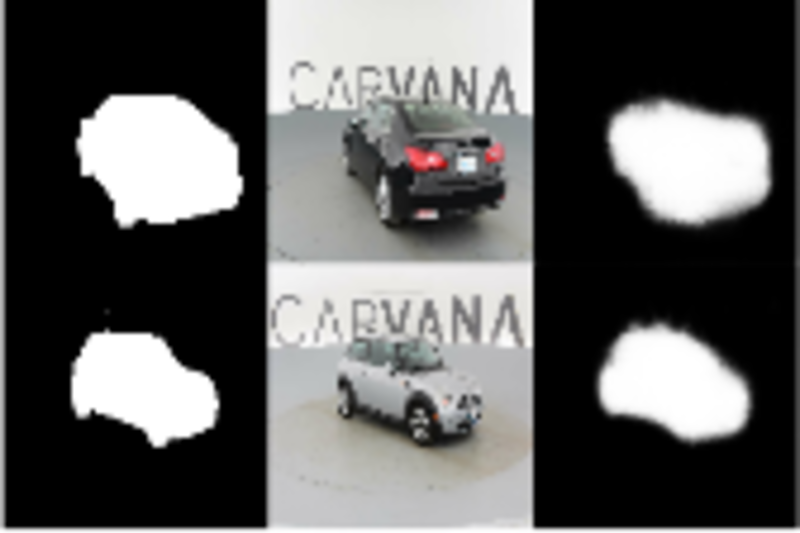Figure 3: From left to right: ground truth, input image, and predicted mask from U-Net.

## Conclusion

If there were a competition for the highest sum of usefulness and architectural transparency, U-Net would certainly be a contender. Without much tuning, it’s possible to obtain decent results. If you’re able to put this model to use in your work, or if you have problems using it, let us know! Thanks for reading!

Ronneberger, Olaf, Philipp Fischer, and Thomas Brox. 2015. “U-Net: Convolutional Networks for Biomedical Image Segmentation.” CoRR abs/1505.04597. http://arxiv.org/abs/1505.04597.

1. Expect up to half an hour on a laptop CPU.

### Reuse

Text and figures are licensed under Creative Commons Attribution CC BY 4.0. The figures that have been reused from other sources don't fall under this license and can be recognized by a note in their caption: "Figure from ...".

### Citation

`Falbel & Keydana (2019, Aug. 23). TensorFlow for R: Image segmentation with U-Net. Retrieved from https://blogs.rstudio.com/tensorflow/posts/2019-08-23-unet/`
```@misc{falbelkeydana2019unet,